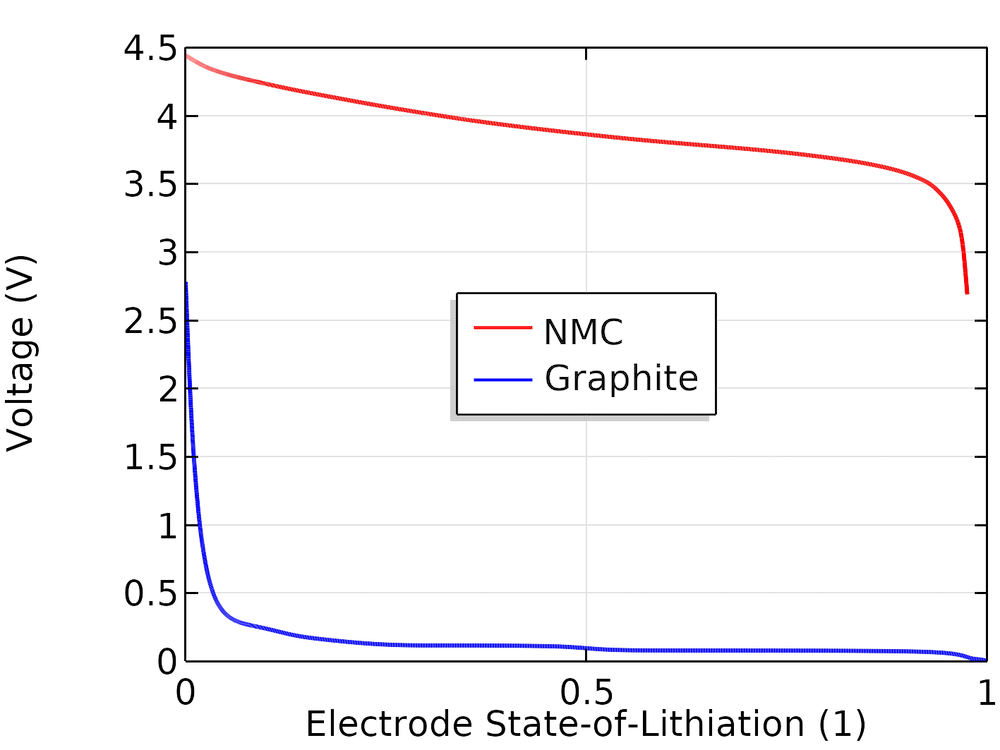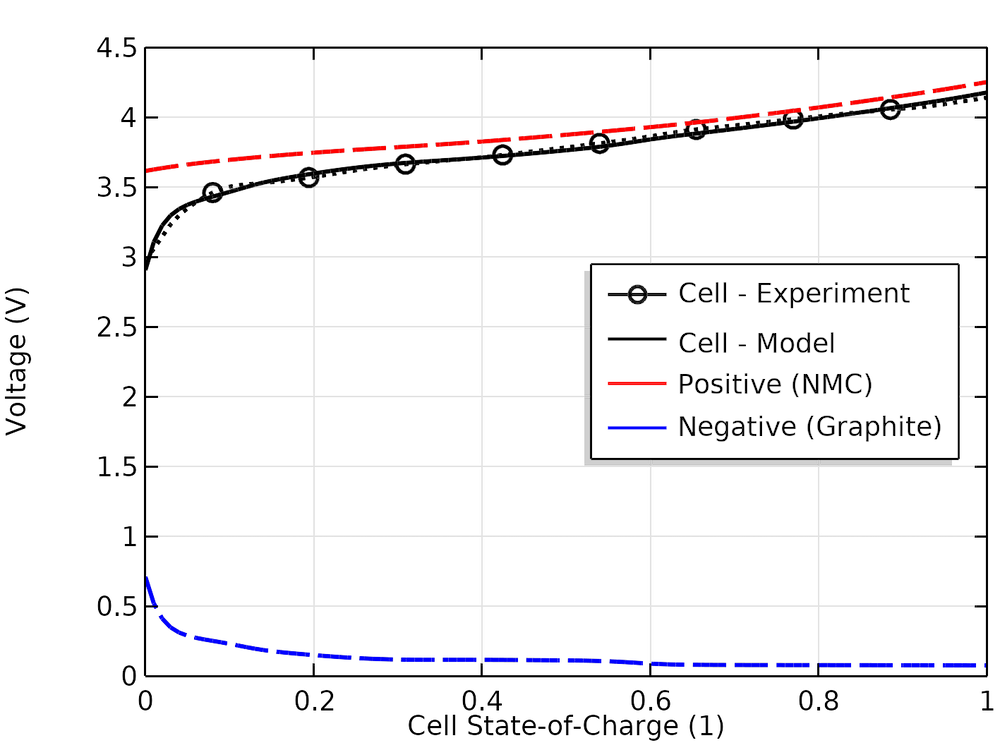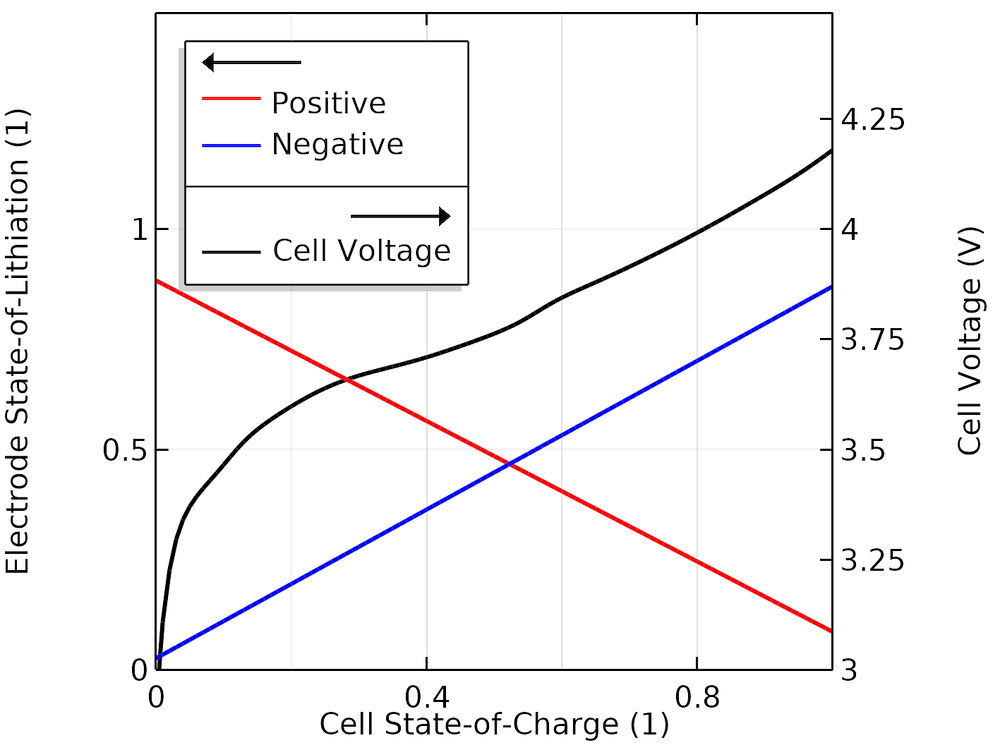# Electrode Balancing of a Lithium-Ion Battery with COMSOL®

May 16, 2019

If the electrode balancing for a lithium-ion battery isn’t right, the cell open-circuit voltage will never be accurate. In this blog post, we introduce electrode balancing and how it can be derived, as well as demonstrate a fitting method to achieve the proper balance for a simple battery model in the COMSOL Multiphysics® software. We also discuss how this method can be used for advanced modeling, such as to predict charge-discharge capacities for different rates and analyze potential-dependent aging reactions.

### Why Is Electrode Balancing Important?

A battery cell consists of one negative (the anode during discharge) and one positive (the cathode during discharge) electrode. In addition, in a lithium cell, the amount of lithium ions being reduced on the cathode needs to be equal to the amount of lithium atoms oxidized on the anode. Balancing the amount of active electrode material on each side is paramount in battery engineering. Having too much of one material means effectively adding deadweight to the battery, since it is not possible to use 100% of that electrode if it is not properly counterbalanced by the other.Think of charging and discharging a battery as pouring an energy drink (lithium) back and forth between two glasses (electrodes). Given that initially, one glass has to be completely filled (lithiated), how should you select the size of each glass to minimize the total container volume while maximizing the amount (capacity) and rate (current) of liquid you can pour without spilling (aging the battery)?

Modeling-wise (for any model defining both the positive and negative electrodes), electrode balancing is of equal importance, as the open-circuit potential of the battery model will not be correct unless a proper balancing is in place. (Also, if you have a bad open-circuit potential, this means your model is inaccurate before you even start running a current through it.)

Let’s start from an engineering perspective to see how we can balance a battery engineered for a certain cell capacity. For this discussion, we will use the following definitions:

• Nominal cell capacity: battery cell capacity for a specified set of maximum and minimum voltage limits at a certain charge/discharge rate
• Host capacity: amount of sites in each electrode where lithium may be stored
• Cyclable lithium: amount (the sum in both electrodes) of cyclable lithium atoms that can be added or removed from the electrodes during cycling

### Electrode Balancing Basics

#### Cyclable Lithium Stems from the Positive Electrode

The negative electrode (graphite, titanate, silicon, etc.) material contains no lithium at manufacture — the material is fully unlithiated — whereas the positive electrode material (a lithium metal oxide, lithium phosphate, etc.) is fully lithiated. The pristine cyclable lithium amount hence equals the host capacity of the positive electrode. A naïve approach for electrode balancing would be to just add as much electrode material on the positive electrode as needed for the battery’s nominal capacity and the corresponding amount of electrode material on the negative. However, it is not that easy.

#### Choosing Capacity on the Positive Electrode

On the positive electrode, high potentials may cause irreversible electrolyte oxidation and gas formation, or the dissolution of metal ions from the lithium oxide host matrix. Full delithiation could also destroy the host matrix. To increase lifetime (or for safety), the battery engineer hence decides to specify a maximum electrode voltage.

This maximum, in essence, means lowering the allowed lithiation window of the positive electrode, which means that more positive electrode host material than the nominal cell capacity has to be used in the cell. (Modeling can be a great tool for assessing the potential levels in the electrode at various charge rates, but that is outside the scope of this blog post.) As a result, we cannot base the electrode balancing solely on the nominal cell capacity, since only a fraction of the positive host capacity may be allowed to be accessed during normal operation. We also need to consider detrimental cycling effects on the negative electrode.

Toward the end of a charge, the negative electrode reaches its lowest potentials. Low potentials at the negative electrode are harmful to the battery, since this may result in accelerated solid-electrolyte-interphase (SEI) formation and because the electrode potential starts to approach the reversible potential for lithium metal plating.

If lithium metal plating occurs in the cell, it may lead to additional loss of the cyclable lithium and also result in the growth of lithium metal dendrites that risk short-circuiting the cell. In addition, there are mechanical issues in electrodes made of graphite at low potentials, due to an increased coefficient of volume expansion when close to 100% lithiation.

The risk of low potentials and close to 100% lithiation locally is further accentuated by uneven current distributions at high charging currents (which modeling can help assess). It is therefore common to add excess amounts of negative electrode host capacity in relation to the nominal capacity of the battery. Things are, however, even more complex.

#### Formation and Aging

The first set of charge-discharge cycles of the cell after manufacturing is called formation. During formation, a small amount of cyclable lithium is lost due to parasitic reactions, for instance, due to reactions creating the initial SEI layer. This loss reduces the amount of lithium available for charge cycling.

During cycling, lithium may also get so tightly bound into the host matrix of one of the electrodes that it is no longer accessible for normal charge-discharge cycling. Also, particle cracking can result in active material getting unconnected from the rest of the electrode. Phenomena of this kind will reduce both the amount of cyclable material and the host capacity of the electrode.

Typically, the amount of irreversibly lost cyclable lithium and host capacity during formation are compensated for when balancing the battery. However, even though the lifetime issues can be minimized with a good balance and by enforcing cycling voltage constraints in the device, they can never be fully mitigated. As the battery ages, cyclable lithium and host material will continuously be lost, resulting in a dynamically changing balancing.

#### Electrode Balancing and Modeling

It is hard to exaggerate the importance of the electrode balancing being right in a model. If your thermodynamics (that is, the open-circuit potentials) are not right, there is simply no way you can compensate for them by tweaking conductivities, exchange current densities, and diffusion coefficients — your cell open-circuit voltage will never be accurate with a bad balancing.

In the following, we will present a mathematical framework for electrode balancing and then use this framework in two different examples.

### Mathematical Framework for Electrode Balancing

Three physical charge parameters are needed to define the cell balancing:

1. Q_\textrm{host,pos}(Ah) — amount of host material in the positive electrode
2. Q_\textrm{host,neg}(Ah) — amount of host material in the negative electrode
3. Q_\textrm{Li,tot}(Ah) — total amount of lithium atoms in both electrodes (available for electrochemical reactions)

For each electrode, the electrode state-of-lithiation (SOL) is defined as

\textrm{SOL}_\textrm{pos} \equiv \frac{Q_\textrm{Li,pos}}{ Q_\textrm{host,pos}}

and

\textrm{SOL}_\textrm{neg} \equiv \frac{Q_\textrm{Li,neg}}{ Q_\textrm{host,neg}}

where Q_\textrm{Li,pos}(Ah) and Q_\textrm{Li,neg}(Ah) are the amount of lithium atoms stored in the positive and negative electrodes, respectively.

We now introduce the dimensionless Y parameters, which are made dimensionless by dividing the corresponding charge parameter Q (Ah) by the nominal capacity of the battery, Qcell:

Y_i = \frac{Q_i}{Q_\textrm{cell}}

To define the cell state-of-charge (SOC), we also need to couple the lithiation level in one of the electrodes to a specified SOC level.

In summary, we need four parameters to define the balancing model:

1. Y_\textrm{host,pos}— dimensionless amount of host material in the positive electrode
2. Y_\textrm{host,neg}— dimensionless amount of host material in the negative electrode
3. Y_\textrm{Li,tot}— dimensionless total amount of lithium
4. \textrm{SOL}_{\textrm{neg},0}— SOL of the negative electrode at 0% cell SOC

The SOLs of the electrodes can now be written as

\textrm{SOL}_\textrm{neg} = \textrm{SOL}_{\textrm{neg},0}+\frac{\textrm{SOC}}{Y_\textrm{host,neg}}

for the negative electrode and

\textrm{SOL}_\textrm{pos} = \frac{Y_\textrm{Li,tot}}{Y_\textrm{host,pos}} – \frac{\textrm{SOL}_{\textrm{neg}}Y_\textrm{host,neg}}{Y_\textrm{host,pos}}

for the positive electrode.

When working with the balancing expressions, it is often useful to keep in mind that the host material parameters relates directly to the difference in lithiation levels between 0 and 100% cell SOC according to

Y_\textrm{host,neg}= \frac{1}{\textrm{SOL}_\textrm{neg,100} – \textrm{SOL}_\textrm{neg,0} }

Y_\textrm{host,pos}= \frac{1}{\textrm{SOL}_\textrm{pos,0} – \textrm{SOL}_\textrm{pos,100} }

The above expressions indicate that if wider SOL cycling windows can be achieved (and allowed) for a given nominal cell capacity, this increases the energy density of the battery by reducing the required amounts of active material in the electrodes.

### Example 1: Choosing an Electrode Balancing from a Battery Cell Engineer’s Point of View

The four electrode balance parameters can be selected in various ways. In this example, we look at the problem from the view of a battery cell engineer and construct a balancing based on a:

• Chosen minimum lithiation level in the negative electrode at 0% SOC, \textrm{SOL}_\textrm{neg,0}(1)
• Chosen minimum lithiation level in the positive electrode at 100% SOC, \textrm{SOL}_\textrm{pos,100}(1)
• Desired relative excess host capacity in the negative electrode, f_\textrm{excess}(1)
• Assumed relative loss of cyclable lithium during formation, f_\textrm{loss}(1)

\textrm{SOL}_\textrm{neg,0} can be used directly in the framework above.

The excess capacity is here defined as the extra host capacity present in the negative electrode (the margin between the lithiation level at 100% SOC and full lithiation) in relation to the capacity accessed during cycling between 0 and 100% SOC; that is:

f_\textrm{excess} \equiv \frac{1-\textrm{SOL}_\textrm{neg,100}}{\textrm{SOL}_\textrm{neg,100} – \textrm{SOL}_\textrm{neg,0}}

which can be rearranged into:

Y_\textrm{host,neg} = \frac{1+f_\textrm{excess}}{1-\textrm{SOL}_\textrm{neg,0}}

Determining Y_\textrm{host,pos} and Y_\textrm{Li,tot} is a bit more tricky. During formation, we first assume that all cyclable lithium is lost due to formation of the initial SEI layer in the negative electrode, and that this capacity loss is proportional to Y_\textrm{host,neg}. We hence write:

f_\textrm{loss} \equiv \frac{Y_\textrm{host,pos}-Y_\textrm{Li,tot}}{Y_\textrm{host,neg}}

which may be rearranged into:

Y_\textrm{Li,tot} = Y_\textrm{host,pos} – f_\textrm{loss}Y_\textrm{host,neg}

Secondly, we use the formulas for \textrm{SOL}_\textrm{pos} and \textrm{SOL}_\textrm{neg} above at 100% SOC to relate \textrm{SOL}_\textrm{pos,100} to the other parameters:

\textrm{SOL}_\textrm{pos,100} = \frac{Y_\textrm{Li,tot}}{Y_\textrm{host,pos}} – \frac{\textrm{SOL}_{\textrm{neg,100}}Y_\textrm{host,neg}}{Y_\textrm{host,pos}} = \frac{Y_\textrm{Li,tot}}{Y_\textrm{host,pos}} – \frac{\left(\textrm{SOL}_{\textrm{neg},0}Y_\textrm{host,neg}+1\right)}{Y_\textrm{host,pos}}

Rearranging results in the following expressions for Y_\textrm{host,pos} and Y_\textrm{Li,tot}:

Y_\textrm{host,pos} = \frac{1+Y_\textrm{host,neg}(f_\textrm{loss}+\textrm{SOL}_{\textrm{neg},0})}{1-\textrm{SOL}_\textrm{pos,100}}

Y_\textrm{Li,tot} = \frac{1+Y_\textrm{host,neg}(f_\textrm{loss}\textrm{SOL}_\textrm{pos,100}+\textrm{SOL}_{\textrm{neg},0})}{1-\textrm{SOL}_\textrm{pos,100}}

### Example 2: Reverse Engineering the Electrode Balance Using COMSOL Multiphysics®

As indicated by the introduction and the first example, quite a lot of information is needed in order to construct a correct electrode balance in a model. If there are any errors in any of the parameters, the open-circuit voltage of the model may deviate significantly from that of a real cell. An additional problem with commercial batteries (that you have not constructed yourself) is that the needed parameters may simply not be known.

In this example, we will use a reverse-engineering approach as an alternative way of electrode balancing, starting from an experimental open-circuit voltage of a cell and some basic assumptions, followed by the use of an optimization solver to find a proper electrode balancing.

The experimental open-circuit voltage of the cell is shown in the figure below. The cell SOC is, in this case, defined as going from 0 to 1 when the open-circuit voltage goes from 3.0 to 4.15 V.Experimental open-circuit voltage versus cell SOC.

The cell is assumed to be a (1:1:1) LiNiMnCo (NMC)-graphite cell. To construct the cell open-circuit potential, we use the corresponding individual electrode equilibrium potential curves available in the material library in the Batteries & Fuel Cells Module, an add-on to COMSOL Multiphysics. These curves are based on experimental half-cell data and represented as interpolation polynomials for the equilibrium potential, Eeq (V), as a function of the state of lithiation (SOL), as shown below.Equilibrium electrode potentials versus SOL.

Using the mathematical framework above, the cell open-circuit potential is then constructed as the difference between the positive and negative open-circuit voltages:

E_\textrm{cell,model} = E_\textrm{eq,pos}(\textrm{SOL}_\textrm{pos}) – E_\textrm{eq,neg}(\textrm{SOL}_\textrm{neg})

Using an optimization solver, we let COMSOL Multiphysics perform a parameter estimation of our four fitting parameters (Y_\textrm{host,pos}, Y_\textrm{host,neg},Y_\textrm{Li,tot}, and \textrm{SOL}_{\textrm{neg},0}), using the method of least squares and minimizing the objective function, O, which is defined as:

O = \sum (E_\textrm{cell,model} – E_\textrm{cell,experiment} )^2

for all of our data points in the experimental open-circuit potential (OCP) curve versus SOC.

#### Results and Discussion

The experimental and modeled cell voltage versus cell SOC together with the individual electrode voltage values are shown below. We can see that the half-cell curves from the material library, which had not been measured for this particular cell, can approximate the true experimental cell OCP fairly well.Experimental and model cell open-circuit voltages versus SOC and corresponding modeled positive and negative electrode voltages.

The table shows the fitted parameter values. The term Y_\textrm{Li,tot} is higher than 1, indicating that all capacity is not used when cycling within the voltage window of the cell OCP curve. Similarly, Y_\textrm{host,neg} being higher than 1 indicates that there is about 16% of excess host capacity at the negative electrode (see definition of f_\textrm{excess} in Example 1) to allow for a potential margin versus lithium plating during charge. We also note that Y_\textrm{Li, tot} is smaller than Y_\textrm{host,pos} by about 10%, giving a measure of the amount of lithium lost during formation (see the definition of f_\textrm{loss} in Example 1).

Parameter Fitted Value

Y_\textrm{host,pos}
1.25

Y_\textrm{host,neg}
1.19

Y_\textrm{Li, tot}
1.14

\textrm{SOL}_{\textrm{neg},0}
0.025

The figure below shows the cell voltage and corresponding SOL values as a function of the cell SOC, indicating the actual SOL cycling windows of the electrodes when cycling between 0 and 100% cell SOC for our fitted model.Modeled SOL in each electrode versus SOC.

The above fitting method is undoubtedly usable for obtaining a proper electrode balancing in a model. However, it can also be used for direct analysis of an aged cell (state of health). Assuming that the shape of the half-cell equilibrium potential curves versus the SOL does not change for aged cells, we can perform the above fitting procedure over time to monitor the state of health of the cell. Changes in the fitted values of Y_\textrm{Li, tot}, Y_\textrm{host,neg}, or Y_\textrm{host,pos} then correspond to a loss of cyclable lithium and/or host material. This can be seen as a complementary method to the frequently used dVdQ approach, where the positions of the peaks in the derivative of the open-circuit voltage versus SOC curves are analyzed instead.

### Using the Cell Balancing Parameters for More Advanced Modeling

Any set of the four electrode balancing parameters could now be used to construct more advanced models; for instance, performance modeling to predict charge-discharge capacities for different rates or detailed modeling of certain potential-dependent aging reactions.

For example, the host parameters above would correspond to the following expressions in the Lithium-Ion Battery interface in COMSOL Multiphysics

Q_\textrm{host, pos}= F \int_\textrm{pos} \varepsilon_s c_{s,\textrm{max}} \textrm{d} \Omega

and

Q_\textrm{host, neg}= F \int_\textrm{neg} \varepsilon_s c_{s,\textrm{max}} \textrm{d} \Omega

where the integral is taken over the positive and negative electrode domains, respectively. Here, \varepsilon_s is the volume fraction of the electrode in the porous domain, and c_{s,\textrm{max}} (mol/m3) is the maximum amount of intercalated lithium; i.e., the volumetric host capacity of the electrode phase.

Assuming the electrode properties to be constant in each electrode, the electrode volume fractions may hence be set as follows:

\varepsilon_{s,\textrm{neg}} = \frac{Y_\textrm{host, neg} Q_\textrm{cell}} {F c_{s,\textrm{max,neg}} L_\textrm{neg} A}

and

\varepsilon_{s,\textrm{pos}} = \frac{Y_\textrm{host, pos} Q_\textrm{cell}} {F c_{s,\textrm{max,pos}} L_\textrm{pos} A}

respectively, where L_\textrm{neg}(m) and L_\textrm{pos} is the thickness of each electrode and A (m2) is the electrode cross-sectional area.

The remaining two parameters relate to the initial values of the intercalated lithium concentrations in the electrodes. In the porous electrode model, the local state of lithiation is defined as:

\textrm{SOL} \equiv \frac{c_s}{c_{s, \textrm{max}}}

where c_s is the local concentration of intercalated lithium.

Expressions for the initial concentrations can hence be derived from the expressions for SOLneg and SOLpos above. For instance, setting the initial concentrations to correspond to a 0% cell SOC as defined above would correspond to

c_{s,\textrm{init},\textrm{neg}} = c_{s,\textrm{max},\textrm{neg}} \textrm{SOL}_{\textrm{neg},0}

in the negative electrode domain and

c_{s, \textrm{init},\textrm{pos}} = c_{s,\textrm{max},\textrm{pos}}\left(\frac{Y_\textrm{Li, tot}}{Y_\textrm{host,pos}} – \frac{\textrm{SOL}_{\textrm{neg},0}Y_\textrm{host,neg}}{Y_\textrm{host,pos}}\right)

in the positive electrode domain.

### Next Steps

To learn about the features and functionality for battery modeling in the Batteries & Fuel Cells Module, click the button below:

• How to Model Short Circuits in Lithium-Ion Batteries
• Li-Ion Battery: Heterogeneous Alternative to the Newman Model
• Evaluating the Electrode Utilization of a Lithium-Ion Battery Pouch Cell
• #### Categories##### Abigail Rozenblit
August 20, 2020

Hello, thank you for this blog post, it is really useful. I would like to ask a question. If you do not have access to a lab (because of the pandemic), and you would like to practice electrode balancing simulations, where would you look for the OCV vs SOC curves? I have been looking online for that information about my system and can’t seem to find it. Maybe COMSOL has some sort of data base for these kinds of curves? Thank you in advance.##### Henrik Ekström
August 20, 2020 COMSOL Employee

Thank you Abigali. I am afraid I cannot disclose were that very curve came from, but it was based on experimental data recorded in a lab. Some battery manufacturers may disclose OCV vs SOC curves on their homepages – otherwise you need connections to a lab.

EXPLORE COMSOL BLOG